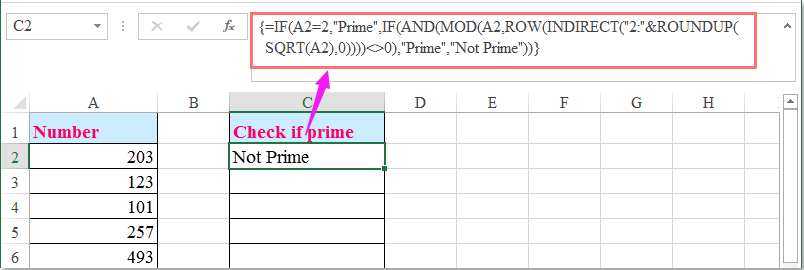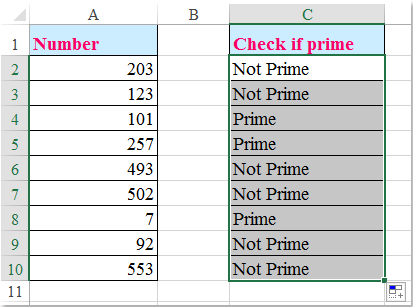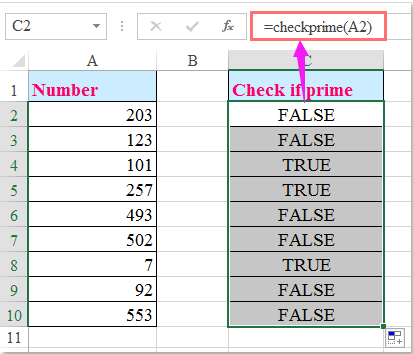orRegisteror

## How to check if the number is prime number in Excel?

As we all known, a prime number is a natural number which contains only two distinct natural number divisors: One and itself. If you have a list of numbers in a worksheet, how could you check if the numbers are prime numbers?

Check if a number is prime number with array formula

Check if a number is prime number with User Defined Function

####Check if a number is prime number with array formula###### Save 50% of your time, and reduce thousands of mouse clicks for you every day!

The following formula can help you identify the number whether it is a prime number or not, please do as this:

1. Enter the following formula into a blank cell – C2 for example beside your data:

=IF(A2=2,"Prime",IF(AND(MOD(A2,ROW(INDIRECT("2:"&ROUNDUP(SQRT(A2),0))))<>0),"Prime","Not Prime")) (A2 is the cell contains the number that you want to check), and then press Ctrl + Shift + Enter keys together, and you will get the result, if the number is prime, it will display “Prime” in the cell, if not, “Not Prime” will be displayed, see screenshot:2. Then select the cell C2, and drag the fill handle down to the cells which you want to apply this formula, and all the numbers will be identified if is prime number or not. See screenshot:####Check if a number is prime number with User Defined Function

The following User Defined Function also can help you to check the numbers if prime or not, please do as follows:

1. Hold down the ALT + F11 keys to open the Microsoft Visual Basic for Applications window.

2. Click Insert > Module, and paste the following code in the Module Window.

VBA code: Check if a number is prime or not:

```Function CheckPrime(Numb As Single) As Boolean
'Updateby Extendoffice 20160614
Dim X As Long
If Numb < 2 Or (Numb <> 2 And Numb Mod 2 = 0) _
Or Numb <> Int(Numb) Then Exit Function
For X = 3 To Sqr(Numb) Step 2
If Numb Mod X = 0 Then Exit Function
Next
CheckPrime = True
End Function
```

3. Then save and close this code, go back to the worksheet, and enter this formula: =checkprime(A2) into a blank cell beside your number list, and then drag the fill handle down to the cells which you want to contain this formula, if the number is prime number, it will display TRUE, if not, it will display FALSE, see screenshot:### The Best Office Productivity Tools

#### Kutools for Excel Solves Most of Your Problems, and Increases Your Productivity by 80%

• Reuse: Quickly insert complex formulas, charts and anything that you have used before; Encrypt Cells with password; Create Mailing List and send emails...
• Super Formula Bar (easily edit multiple lines of text and formula); Reading Layout (easily read and edit large numbers of cells); Paste to Filtered Range...
• Merge Cells/Rows/Columns without losing Data; Split Cells Content; Combine Duplicate Rows/Columns... Prevent Duplicate Cells; Compare Ranges...
• Select Duplicate or Unique Rows; Select Blank Rows (all cells are empty); Super Find and Fuzzy Find in Many Workbooks; Random Select...
• Exact Copy Multiple Cells without changing formula reference; Auto Create References to Multiple Sheets; Insert Bullets, Check Boxes and more...
• Extract Text, Add Text, Remove by Position, Remove Space; Create and Print Paging Subtotals; Convert Between Cells Content and Comments...
• Super Filter (save and apply filter schemes to other sheets); Advanced Sort by month/week/day, frequency and more; Special Filter by bold, italic...
• Combine Workbooks and WorkSheets; Merge Tables based on key columns; Split Data into Multiple Sheets; Batch Convert xls, xlsx and PDF...
• More than 300 powerful features. Supports Office/Excel 2007-2019 and 365. Supports all languages. Easy deploying in your enterprise or organization. Full features 30-day free trial. 60-day money back guarantee.#### Office Tab Brings Tabbed interface to Office, and Make Your Work Much Easier

• Enable tabbed editing and reading in Word, Excel, PowerPoint, Publisher, Access, Visio and Project.
• Open and create multiple documents in new tabs of the same window, rather than in new windows.
• Increases your productivity by 50%, and reduces hundreds of mouse clicks for you every day!Say something here...
symbols left.
###### or post as a guest, but your post won't be published automatically.
• To post as a guest, your comment is unpublished.
· 5 days ago
Hi.

I am using an italian version of Excel, so the formula does not work and i have an error. Can you help me?

• To post as a guest, your comment is unpublished.
· 4 days ago
Hi, Gian,
If the formula does not work correctly for you, you can apply the second method-User Defined Function. Please try, hope it can help you!
Thank you!
• To post as a guest, your comment is unpublished.
· 6 months ago
Yes it worked wen I did Ctrl+Shift+Enter... !! Thank you..:D and Do u mind explaining the algorithm please.
• To post as a guest, your comment is unpublished.
· 8 months ago
There's a reference error when you type in numbers that have more than 12 digits.
• To post as a guest, your comment is unpublished.
· 7 months ago
Hello,Nathan,
As you said, when the numbers are longer than 12 digits, it will become scientific notation. The formula is not applied for this formatting.
• To post as a guest, your comment is unpublished.
· 1 years ago
well 4095 is not a prime. still it gives result as prime no though. its incorrect.
• To post as a guest, your comment is unpublished.
· 1 years ago
Hello, vaibhav,
When you pasting above formula, you should press Ctrl + Shift + Enter keys together, not just Enter key, please try it again.
• To post as a guest, your comment is unpublished.
· 2 years ago
Your formula is not working. Eg. 1681 is NOT a prime but by formula it is.
=IF(A2=2,"Prime",IF(AND(MOD(A2,ROW(INDIRECT("2:"&ROUNDUP(SQRT(A2),0))))<>0),"Prime","Not Prime"))
• To post as a guest, your comment is unpublished.
· 2 years ago
Hello, Jan,
The above formula is correct, after pastingt the formula into a cell, you should press Ctrl + Shift + Enter keys together, not just Enter key.
Please try it again, thank you!
• To post as a guest, your comment is unpublished.
· 2 years ago
[b]this is my fastest version[/b]

starting_number = 1 'input value here
last_number = 30000 'input value here
For a = starting_number To last_number
c = a
For b = 2 To c
If a Mod b = 0 And c b Then
Exit For
Else
If b = c Then
Exit For
Else
If Round(a / (b + 1)) + 1 > b Then
c = Round(a / (b + 1)) + 1
End If
End If
End If
Next b
Next a
End Sub

Sub ISPRIME3()
number_to_be_checked = 2000000000 'input value here
c = number_to_be_checked
For b = 2 To c
If number_to_be_checked Mod b = 0 And c b Then
MsgBox "Not Prime. Divisible by " & b
Exit Sub
Else
If b = c Then
MsgBox "Prime"
Exit Sub
Else
If Round(number_to_be_checked / (b + 1)) + 1 > b Then
c = Round(number_to_be_checked / (b + 1)) + 1
End If
End If
End If
Next b
End Sub
• To post as a guest, your comment is unpublished.
· 2 years ago
The user defined function does not seem to work for numbers above ~16777213
• To post as a guest, your comment is unpublished.
· 2 years ago
starting_number = 99990 'input value here
last_number = 99999 'input value here
For a = starting_number To last_number
For b = 2 To a
If a - b * Int(a / b) = 0 And a b Then
Exit For
Else
If a = b Then
End If
End If
Next b
Next a
End Sub

For a = starting_number To last_number
For b = 2 To a
If a - b * Int(a / b) = 0 And a b Then
Exit For
Else
If a = b Then
End If
End If
Next b
Next a
End Function

'to check if a number is prime
Sub ISPRIME()
number_to_be_checked = 102 'input value here
For b = 2 To number_to_be_checked
If number_to_be_checked - b * Int(number_to_be_checked / b) = 0 And _
number_to_be_checked b Then
MsgBox "Not Prime. Divisible by " & b
Exit Sub
Else
If number_to_be_checked = b Then
MsgBox "Prime"
End If
End If
Next b
End Sub

'to check if a number is prime
Function ISPRIME2(number_to_be_checked)
For b = 2 To number_to_be_checked
If number_to_be_checked - b * Int(number_to_be_checked / b) = 0 And _
number_to_be_checked b Then
ISPRIME2 = "Not Prime. Divisible by " & b
Exit Function
Else
If number_to_be_checked = b Then
ISPRIME2 = "Prime"
End If
End If
Next b
End Function
• To post as a guest, your comment is unpublished.
· 2 years ago
The array formula doesn't work but the User defined function does and was very helpful. Thanks!
• To post as a guest, your comment is unpublished.
· 2 years ago
The array formula above doesn't work but the User Defined function does and was very helpful.Thanks!
• To post as a guest, your comment is unpublished.
· 3 years ago
Hi there,
I like your article. Thanks a lot.
My simple tests showed that 99 was a prime number while 99 is not as 99 is divided by 3 and 33 as well as 1 and 99.
Can you let me know what is wrong with my operation?